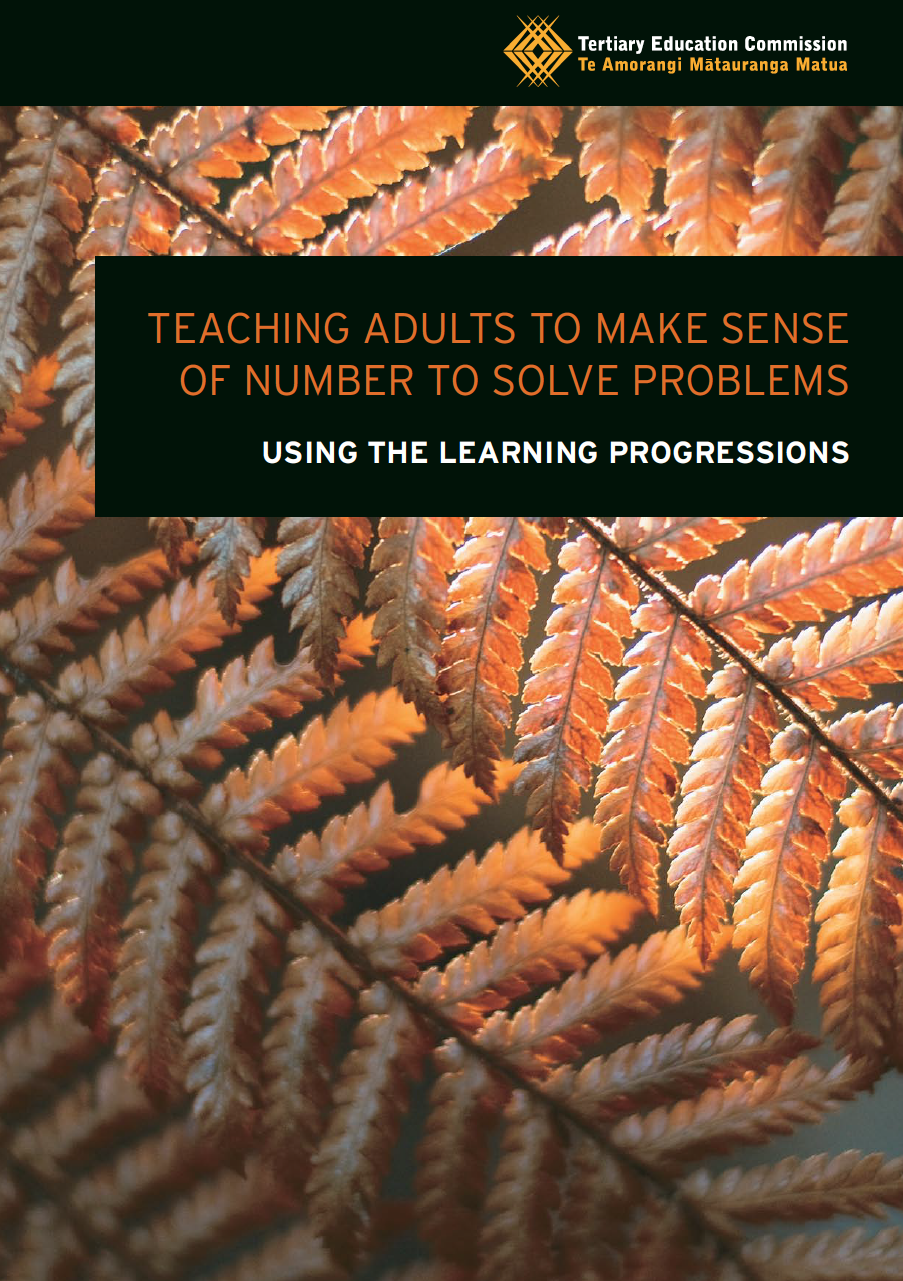# Teach: Cheat sheet for number *strategies* activities from the Learning ProgressionsThis is part one of two parts. Here we just look at numeracy activities that relate to Number strategies. This includes:

• Multiplicative strategies
• Proportional reasoning strategies

You can find numeracy activities that relate to Number Knowledge on a different page.

These resources are filled with activities and ideas that you can use or adapt. But navigating them can be tricky. So we designed a cheat sheet.

# Cheat Sheet for Number Strategies Activities

Here’s how to use it. Just look down the list at the second column. This tells you in one sentence what learners actually have to do in the activity.

From there, you can look at the name of the activity, find the page number in the resource, and see which progressions it lines up with.

Things to remember:

• Most activities cut across multiple progressions. This is fine. Just make sure you know what your focus is. Refer back to your learning outcomes.
• These numeracy activities are specific to certain steps. Make sure that you know what step your learners are at first.
• Not all the activities will be useful to you and your situation. If you do decide to take the time to look through these, you might want to rate them for yourself. In other words, can you use this?
• You don’t have to use these. If you have your own ideas then go with that. But remember, if you need inspiration or want to look at activities that were written by experts then look here.

You can download the cheat sheets for all three strategy progressions as a single PDF below or just read on.

## Additive Strategies Activity Cheat Sheet

 Activity What do learners do? Additive strategies Where do I find it? Can I use it? Rate: ✓ ? ✗ Counting on and back Count on in ones to solve problems; Learn that you don’t have to start at number one Step 2 P. 19 Addition and subtraction strategies I Use number lines to develop addition and subtraction partitioning strategies for two-digit by one-digit problems Step 3 P. 22 Addition and subtraction strategies II Use number lines to develop addition and subtraction partitioning strategies for multi-digit problems Step 4 P. 25 Adding decimals Use strategies, traditional written methods and calculators to solve addition problems using decimals. Step 5 P. 28 Subtracting decimals Use strategies, traditional written methods and calculators to solve subtraction problems using decimals. Step 5 P. 30

## Multiplicative Strategies Activity Cheat Sheet

#### Can I use it? Rate: ✓ ? ✗

Skip counting Skip count in twos, threes, fives to solve simple problems; Begin to learn the 2, 3, and 5 times tables. Step 2 P. 32
Understanding multiplication Identify unknown multiplication facts; Use known facts to develop recall of unknown facts Step 3 P. 35
Deriving multiplication and division facts Use already known facts to derive and extend knowledge of multiplication facts they need for quick recall Step 3 P. 37
Multiplication strategies Develop mental strategies for solving multiplication problems with single-digit multipliers Step 4 P. 39
Division strategies Develop mental strategies for solving division problems with single-digit divisors Step 4 P. 41
Multiplying options Use strategies, traditional written methods and calculators to solve multiplication problems. Step 5 P. 43
Dividing options Use strategies, traditional written methods and calculators to solve division problems. Step 5 P. 46
Multiplying with decimals Use estimation strategies and calculators to solve multiplication problems using decimals Step 6 P. 49
Dividing with decimals Use estimation strategies and calculators to solve division problems using decimals Step 6 P. 51

## Proportional Reasoning Strategies Activity Cheat Sheet

#### Can I use it? Rate: ✓ ? ✗

Ratios I Develop an understanding of simple ratios; learn to identify ratios for given quantities and vice versa Step 4 P. 53
Fractions of numbers I Develop an understanding of how to find a fraction of a whole number where the answer is also a whole number Step 4 P. 55
Ratios II Develop an understanding of more complex ratios Step 5 P. 58
Fractions of numbers II Develop an understanding of how to find a fraction of a whole number where the answer is also a fraction Step 5 P. 61
Rates and proportions Apply understanding of ratios to rates and proportions Step 6 P. 64## Author: Graeme Smith

Education, technology, design. Also making cool stuff...